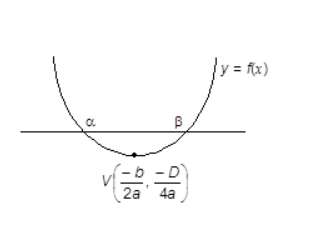# The real numberfor which the equation,   has two distinct real roots inOption 1) does not exist. Option 2) lies between 1 and 2 . Option 3) lies between 2 and 3 . Option 4) lies between -1 and 0 .

N neha
H Himanshu

As we have learned

Quadratic Expression Graph when a> 0 & D > 0 -

Real and distinct roots of

&

- whereinis the abscissa of vertex

and , it should lie in(0,1 ) but it's not true

S, no value of 'k' exists

Option 1)

does not exist.

Option 2)

lies between 1 and 2 .

Option 3)

lies between 2 and 3 .

Option 4)

lies between -1 and 0 .

Exams
Articles
Questions